# Sedimentation Tank Design ParametersReading time: 1 minute

Sedimentation is the process of removing suspended coarser particles in water by settling down them to the bottom of tank. For a particle to settle down, the flow velocity must be reduced. This process is carried out in a structure called sedimentation tank or settling tank.

## Working Principle of Sedimentation Tank

The main principle involved in the sedimentation tank is to reduce the flow velocity of water which allows the major amount of suspended particles to settle down. The velocity with which the particle is settling is known settling velocity.The amount of suspended particles collected at bottom of the tank is based on different factors like shape and size of tank, size of particle, temperature of water, flow velocity, detention period etc.The settling velocity of the particle "Vs " is calculated by Stoke’s law which was obtained from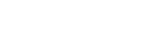Where Vs = settling velocity of particle g = acceleration due to gravity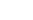= Mass density of water and particle d = diameter of particle Cd = drag coefficient and it is obtained by knowing the Reynolds’s number (R) For laminar flow, Cd = 24/R For transitional flow,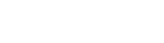For turbulent flow, Cd = 0.4 Where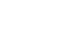If diameter of the particle is less than 0.1 mm then it is considered as laminar flow. Hence for laminar flow equation for settling velocity of the particle after substituting drag coefficient is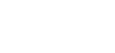We know specific gravity of particle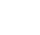and unit weight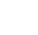Therefore,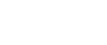Similarly when diameter of the particle is more than 0.1 mm then it is considered as turbulent flow then equation for settling velocity is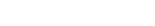## Sedimentation Tank Design Parameters

To design Sedimentation tank following elements are required in the consideration:
• Over flow velocity
• Detention period
• Flow through velocity
• Dimensions of the tank
• Sludge zone depth
• Efficiency

### Overflow Velocity (Vo)

The volume of water applied per unit time per unit horizontal surface area is called over flow velocity. It is also called as surface loading rate or surface over flow rate. Over flow velocity must be less than settling velocity of particle otherwise the particle or sediment will escapes without settling. The over flow velocity is calculated from the formula

Vo = Discharge / surface area = Q / (BxL)

In general, over flow velocity of sedimentation tank = 12 to 18 m3/day/m2.### Flow Through Velocity (V)

The velocity of water with which it travels from inlet to outlet of sedimentation tank is called flow through velocity (V). The allowable flow through velocity of water in sedimentation tank is 0.005m/s.

Flow through velocity, V = discharge / area = Q /(BxH)### Detention Period (T)

The time taken by water to travel from inlet to outlet is termed as detention period or detention time. The allowable detention period in the sedimentation tank is 4 to 8 hours.

Detention Time = volume of tank / discharge = V/Q### Dimensions of Sedimentation Tank

The basin dimensions length, breadth and depth of tank are derived from the volume of the tank and over flow velocity. First we have to compute the area of tank,

Area (A) = Volume of water/ over flow velocity

After obtaining the area assume length and depth based on allowable limits as followed below. The breadth of sedimentation tank should be provided is 10 to 12 meters while the length of sedimentation tank should be at least 4 times the breadth of sedimentation tank. The depth of tank should be 3 to 4.5 meters.### Sludge Zone Depth

Sludge zone depth is used to collect the sludge which is nothing but settled particles. It is provided only when removal of the sludge takes place manually. Generally it is limited to 0.8 to 1.2 meters.### Efficiency

Efficiency is nothing but the performance of sedimentation tank. It is maximum when the maximum amount of suspended particles in the raw water are separated. In sedimentation tanks, efficiency purely depends upon the settling velocity and over flow velocity.

Efficiency of Sedimentation Tank (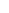) = (Vs/Vo) x 100